# Very simple Arduino capacitance meter using 16 bit timer and analog comparator

As You could see from video it requires only one external resistor. Meter is very simple, but obviously not very accurate or wide range. Theoretically it could measure in 10 nF – 160 µF range.

How it Works?

Circuit formed from resistor and capacitor (RC circuit) has time constant, it shows time needed to discharge capacitor via resistor to ~37% it’s initial voltage. Calculation of time constant is very simple t=R*C . For 1 k resistor and 47µF capacitor it’s 47ms.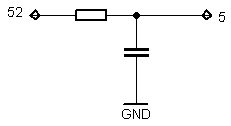My capacitance meter fully charges capacitor, when 16 bit timer is started and capacitor is discharging via resistor (1 k). Capacitor is connected to analog voltage comparator(pin 5), as capacitor voltage drops bellow 1.1 V occurs analog comparator interrupt which triggers timer interrupt.  If resistor is constant, discharge time and capacitance dependency is linear, therefore if You know one rated capacity capacitor discharge time, You could easily calculate another capacitor’s capacitance by measuring time.

Because timer uses only one  /64 clock prescaler measurement range is very narrow. To have wider range there is a always way to make  programmable prescaler, which changes if capacitor value is out of range, or discharge capacitor via range of different resistors.

Also keep in mind that resistor’s resistance depends on environment temperature, consequently and on LCD display showed capacitance. To fix this temperature dependency referenced capacitor should be used. In this case every time both capacitors are charged and discharged separately, but via the same resistor. Capacitance proportion is calculated and if referenced capacitor capacitance is know measured capacitor’s value can be calculated just by division or multiplying.

It’s time for program’s code.

# Arduino 4 digits 7 segments LED countdown timer with buzzer

After sorting out  how works 16 bit hardware timer it is time for 4 digits countdown timer. Having 16 bit timer and  7 segments LED code from earlier only were remaining to write timer’s modes (run/setup) and button’s control code.  After putting  all code to one place there is countdown timer with properties below:

• Maximum 99 minutes 59 seconds countdown interval
• 1 second resolution
• Sound indication with buzzer for finished countdown
• LED indicates running timer, or relay instead  for powering external devices for some period of time
• 2 buttons to set timer and start/pause/reset.

This time without additional code quotation, please find some code explanation within code, so code bellow.

# Examples of using Arduino/Atmega 16 bit hardware timer for digital clock

Arduino Mega with Atmega 1280 has four 16 bit timers, that could be used for various purposes, like time/frequency measurement, control with precise timing, PWM generation. Today I hope to explain how to use timer for clocks, timers (countdown) and other things, where You need µCPU to perform some tasks after precise period of time. I’ll give You two examples:

• Pseudo 1 second timer
• Real 1 second timer

How counter works? It is simple independent  16 bit accumulator, which value increases by 1 at clock cycle. 16 bit means that maximum counter’s value is 65536. When this value is reached counter starts counting from 0 again and gives hardware interrupt. Counter value could be changed any time. This is normal counter mode, Atmega 1280 offers total 14 operating modes.

Pseudo 1 second timer (1.048576 s)

# 4 digits, 7 segments LED display multiplexing with Arduino

Last time I showed You how to control 1 digit 7 segment LED display with Arduino. This time it’s not 1, but 4 digits. To connect 1 digit to Arduino we had to use 8 ports, so to connect 4 digits we need to have 4×8=32? Not necessary. Where is a way to use much less ports, it’s called multiplexing. Using multiplexing at one time only one digit is active(e.g. for 2ms). All digits is turned on is serial, but because human’s eye is inert we have illusion, that all digits are lighting at same time.

As You can see form schematic bellow with multiplexing implemented we required only 4 additional ports compared to 1 digit circuit, total – 12.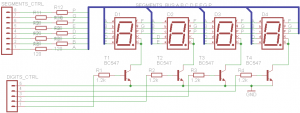Because at the same time only one digit will be on All 4 digits segments inputs are connected together. By connecting digits common cathodes to ground we are controlling which digit shall be turned on. Atmega 1280 µCPU port can drain(receive) maximum 40 mA current. If all one digits segments are on, we are having 20×8= 160 mA that is to much, so we can’t to connect common cathodes directly to Arduino ports.  Therefore I have used BC547 NPN transistors as switches. Transistor is opened, when positive voltage is applied at the base.

Let’s see the code.

# Controlling 7 segment LED display from Arduino. Full code included.

So this is first real electronics mini project/tutorial in this blog.

Everybody at the beginning usually shows how to turn on one LED,  I do it a little more complex by controlling 7 segment LED display.

For this demonstration I use one Kingbright SC56-11EWA module with common cathode, it means that all 8 (7 segments +  1 dot) LED’s cathode are connected together: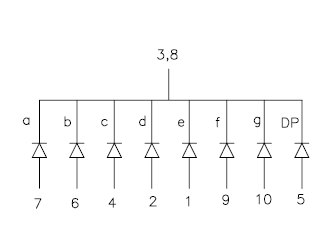The first step is to connect 7 segment display LED’s anodes to Arduino ports. You need 7 free ports, or 8 if You want use “dot”. Display datasheet comes very handy, when You need to found out which pin is which.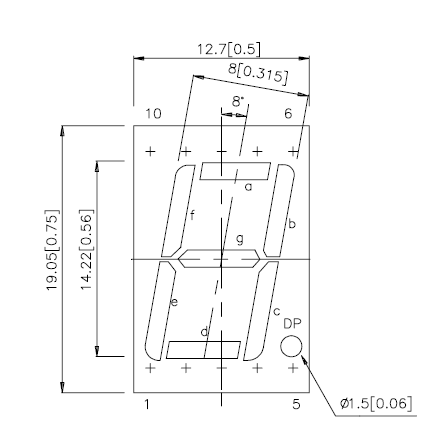Do not forget to connect LED’s pins with resistors in serial. Typical red LED forward voltage is 2.0V @20mA current, but Arduino ports outputs 5V, so You must use resistor, in this situation (5-2)/0.02= 150 Ohm or higher should be ok.

Common cathode (3 and/or 8 pin) should be connected to GND and if You have display with common anode You should connect it to +5V.

So if everything is connected we should start to analyze program code.

To make life easier I have done same definitions, so after that I don’t need to remember which Arduino ports I selected to connect display’s segments:

```#define G 22 #define F 23 #define A 24 #define B 25 #define E 26 #define D 27 #define C 28 #define DP 29```

Arduino ports can be outputs (e.g. for controlling LED) or inputs (e.g. to detect pressed button, sense voltage). We need outputs to turn on LED’s so:

```pinMode(A, OUTPUT); pinMode(B, OUTPUT); pinMode(C, OUTPUT); pinMode(D, OUTPUT); pinMode(E, OUTPUT); pinMode(F, OUTPUT); pinMode(G, OUTPUT); pinMode(DP, OUTPUT);```

To display digit on display we need to turn on some segments accordingly to digit.

For example for “7” we need to turn on A B C segments and other segments should be turned off.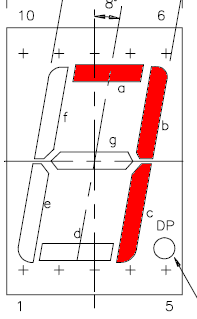So where is function for what as and for all other digits:
`void digit7 () {`

` `

```digitalWrite(A,HIGH); digitalWrite(B, HIGH); digitalWrite(C, HIGH); digitalWrite(D, LOW); digitalWrite(E, LOW); digitalWrite(F, LOW); digitalWrite(G, LOW); }; ```

I also have made a function to display digit form variable, it is very useful in main loop.

```void showdigit (int digit) { switch (digit) { ``````case 0: digit0 (); break; ... case 9: digit9 (); break; default: break; };```

And  the main program. Using for loop as counter from 0 to 9. You see how `showdigit` function is good here, it shows variable “i” content on display.

```for (int i=0;i<10;i++) { //counting from 0 to 9 showdigit(i); delay (1000); // 1000ms= 1s delay if (i%2) { digitalWrite(DP, HIGH); } else {digitalWrite(DP, LOW); };```

If condition is here to check if variable “i” is even and if so shows dot on the display.

Completed code: